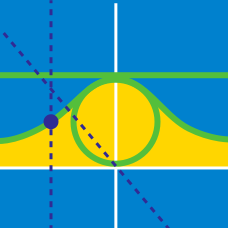Calculus

# Parametric Equations - Tangent

Consider the polar curve $$r\theta = 6$$. What is the slope of the tangent line of the polar curve at $$\theta = \pi$$?

Given the curve $x=1-2t, y=5-4t+2t^2,$ what is the equation of the tangent line to the curve at $$x=5 ?$$

For the curve given by $x=\frac{9t}{1+t^2}, y=\frac{1-t^2}{1+t^2},$ what is the equation of the tangent line to the curve for $$t=2 ?$$

Consider the polar curve $$r = 22 \sin \theta$$. What is the slope of the tangent line at $$\theta = \frac{\pi}{6}$$?

A curve is defined by $$(x,y) =\left(\cos^3 \theta, \sin^3 \theta \right)$$. If the equation for the tangent line at $$\theta=\frac{2}{3}\pi$$ is $$y=ax+b$$, what is the value of $$a \times b$$?

×